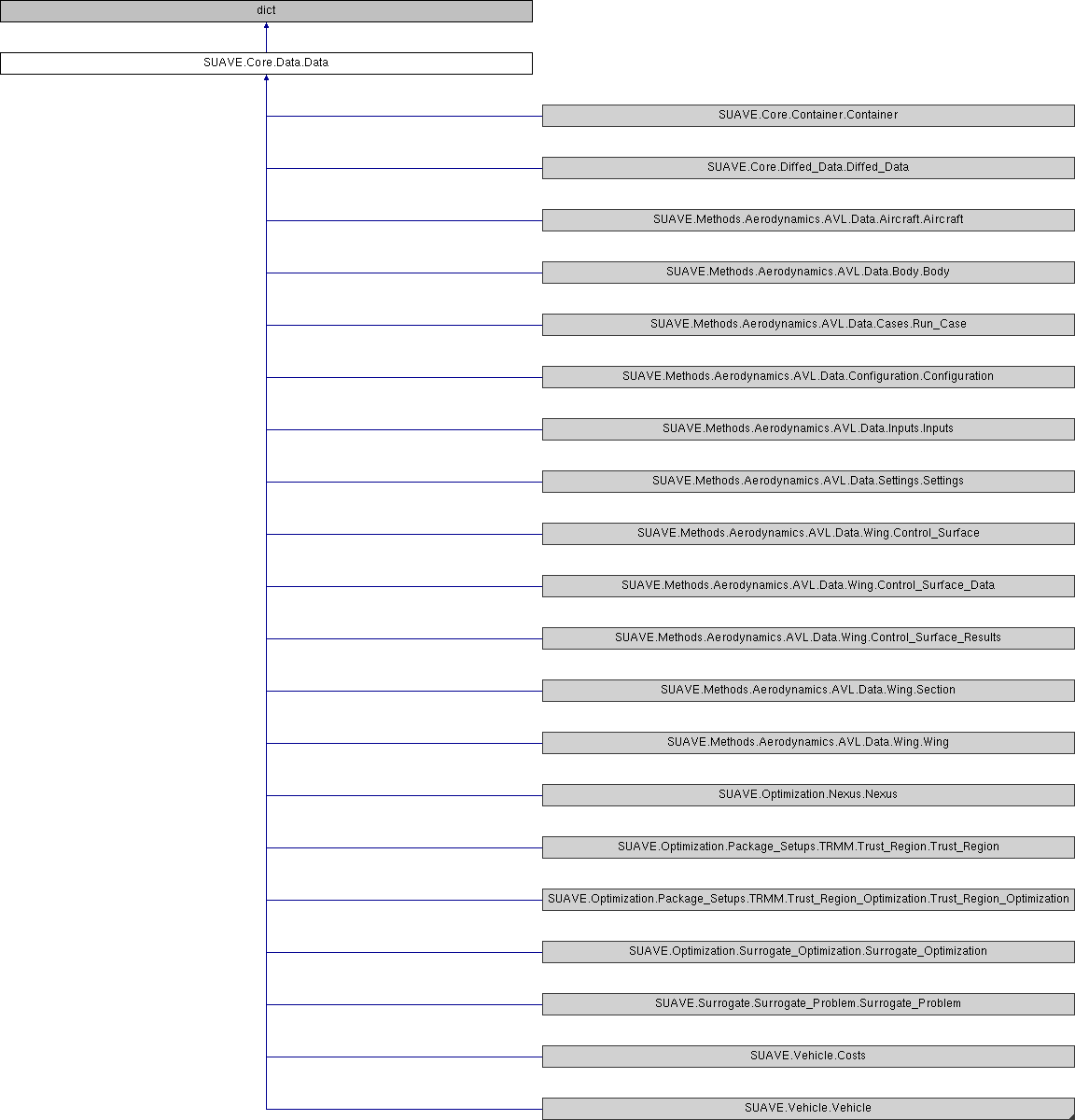SUAVE  2.5.2 An Aerospace Vehicle Environment for Designing Future AircraftSUAVE.Core.Data.Data Class Reference
Inheritance diagram for SUAVE.Core.Data.Data:## Public Member Functions

def __getattribute__ (self, k)

def __setattr__ (self, k, v)

def __delattr__ (self, k)

def __defaults__ (self)

def __new__ (cls, *args, **kwarg)

def typestring (self)

def dataname (self)

def __str__ (self, indent='')

def __init__ (self, *args, **kwarg)

def __iter__ (self)

def itervalues (self)

def values (self)

def update (self, other)

def append_or_update (self, other)

def get_bases (self)

def append (self, value, key=None)

def deep_set (self, keys, val)

def deep_get (self, keys)

def pack_array (self, output='vector')

def unpack_array (self, M)

def do_recursive (self, method, other=None, default=None)

## Detailed Description

```An extension of the Python dict which allows for both tag and '.' usage.
This is an unordered dictionary. So indexing it will not produce deterministic results.
This has less overhead than ordering. If ordering is needed use DataOrdered().

Assumptions:
N/A

Source:
N/A
```

## ◆ __init__()

 def SUAVE.Core.Data.Data.__init__ ( self, * args, ** kwarg )
```Initializes a new Data() class

Assumptions:
N/A

Source:
N/A

Inputs:
N/A

Outputs:
N/A

Properties Used:
N/A
```

Reimplemented in SUAVE.Vehicle.Vehicle, and SUAVE.Core.Container.Container.

## ◆ __defaults__()

 def SUAVE.Core.Data.Data.__defaults__ ( self )
```A stub for all classes that come later

Assumptions:
N/A

Source:
N/A

Inputs:
N/A

Outputs:
N/A

Properties Used:
N/A
```

## ◆ __delattr__()

 def SUAVE.Core.Data.Data.__delattr__ ( self, k )
```An override of the standard __delattr_ in Python. This deletes whatever is called by k

Assumptions:
This one tries to treat k as an object, if that fails it treats it as a key.

Source:
N/A

Inputs:
N/A

Outputs:
N/A

Properties Used:
N/A
```

## ◆ __getattribute__()

 def SUAVE.Core.Data.Data.__getattribute__ ( self, k )
```Retrieves an attribute set by a key k

Assumptions:
Does a try if it is a dict, but if that fails treats it as an object

Source:
N/A

Inputs:
k

Outputs:
whatever is found by k

Properties Used:
N/A```

## ◆ __iter__()

 def SUAVE.Core.Data.Data.__iter__ ( self )
```Returns all the iterable values. Can be used in a for loop.

Assumptions:
N/A

Source:
N/A

Inputs:
N/A

Outputs:
N/A

Properties Used:
N/A
```

## ◆ __new__()

 def SUAVE.Core.Data.Data.__new__ ( cls, * args, ** kwarg )
```Creates a new Data() class

Assumptions:
N/A

Source:
N/A

Inputs:
N/A

Outputs:
N/A

Properties Used:
N/A
```

## ◆ __setattr__()

 def SUAVE.Core.Data.Data.__setattr__ ( self, k, v )
```An override of the standard __setattr_ in Python.

Assumptions:
This one tries to treat k as an object, if that fails it treats it as a key.

Source:
N/A

Inputs:
k        [key]
v        [value]

Outputs:
N/A

Properties Used:
N/A
```

## ◆ __str__()

 def SUAVE.Core.Data.Data.__str__ ( self, indent = `''` )
```This function is used for printing the class. This starts the first line of printing.

Assumptions:
N/A

Source:
N/A

Inputs:
N/A

Outputs:
N/A

Properties Used:
N/A
```

Reimplemented in SUAVE.Core.Diffed_Data.Diffed_Data.

## ◆ append()

 def SUAVE.Core.Data.Data.append ( self, value, key = `None` )
```Adds new values to the classes. Can also change an already appended key

Assumptions:
N/A

Source:
N/A

Inputs:
value
key

Outputs:
N/A

Properties Used:
N/A
```

## ◆ append_or_update()

 def SUAVE.Core.Data.Data.append_or_update ( self, other )
```Appends an array or updates the internal values of a dictionary with given data

Assumptions:
N/A

Source:
N/A

Inputs:
other

Outputs:
N/A

Properties Used:
N/A
```

## ◆ dataname()

 def SUAVE.Core.Data.Data.dataname ( self )
```This function is used for printing the class

Assumptions:
N/A

Source:
N/A

Inputs:
N/A

Outputs:
N/A

Properties Used:
N/A
```

## ◆ deep_get()

 def SUAVE.Core.Data.Data.deep_get ( self, keys )
```Regresses through a list of keys to pull a specific value out

Assumptions:
N/A

Source:
N/A

Inputs:
keys  - The keys to iterate over

Outputs:
value - The value to be retrieved

Properties Used:
N/A
```

## ◆ deep_set()

 def SUAVE.Core.Data.Data.deep_set ( self, keys, val )
```Regresses through a list of keys the same value in various places in a dictionary.

Assumptions:
N/A

Source:
N/A

Inputs:
keys  - The keys to iterate over
val   - The value to be set

Outputs:
N/A

Properties Used:
N/A
```

## ◆ do_recursive()

 def SUAVE.Core.Data.Data.do_recursive ( self, method, other = `None`, default = `None` )
```Recursively applies a method of the class.

Assumptions:
N/A

Source:
N/A

Inputs:
method  - name of the method to access

Outputs:
Result  - the results of the method

Properties Used:
N/A
```

## ◆ get_bases()

 def SUAVE.Core.Data.Data.get_bases ( self )
```Finds the higher classes that may be built off of data

Assumptions:
N/A

Source:
N/A

Inputs:
N/A

Outputs:
klasses

Properties Used:
N/A
```

## ◆ itervalues()

 def SUAVE.Core.Data.Data.itervalues ( self )
```Finds all the values that can be iterated over.

Assumptions:
N/A

Source:
N/A

Inputs:
N/A

Outputs:
N/A

Properties Used:
N/A
```

## ◆ pack_array()

 def SUAVE.Core.Data.Data.pack_array ( self, output = `'vector'` )
```maps the data dict to a 1D vector or 2D column array

Assumptions:
will only pack int, float, np.array and np.matrix (max rank 2)
if using output = 'matrix', all data values must have
same length (if 1D) or number of rows (if 2D), otherwise is skipped

Source:
N/A

Inputs:
output - either 'vector' (default), or 'array'
chooses whether to output a 1d vector or
2d column array

Outputs:
array - the packed array

Properties Used:
N/A  ```

## ◆ typestring()

 def SUAVE.Core.Data.Data.typestring ( self )
```This function makes the .key.key structure in string form of Data()

Assumptions:
N/A

Source:
N/A

Inputs:
N/A

Outputs:
N/A

Properties Used:
N/A
```

## ◆ unpack_array()

 def SUAVE.Core.Data.Data.unpack_array ( self, M )
```unpacks an input 1d vector or 2d column array into the data dictionary
important that the structure of the data dictionary, and the shapes
of the contained values are the same as the data from which the array was packed

Assumptions:
N/A

Source:
N/A

Inputs:
M - either a 1D vector or 2D column array

Outputs:
a reference to self, updates self in place

Properties Used:
N/A
```

## ◆ update()

 def SUAVE.Core.Data.Data.update ( self, other )
```Updates the internal values of a dictionary with given data

Assumptions:
N/A

Source:
N/A

Inputs:
other

Outputs:
N/A

Properties Used:
N/A
```

## ◆ values()

 def SUAVE.Core.Data.Data.values ( self )
```Returns all values inside the Data() class.

Assumptions:
N/A

Source:
N/A

Inputs:
N/A

Outputs:
values

Properties Used:
N/A
```

The documentation for this class was generated from the following file:
• /Users/emiliobotero/Dropbox/SUAVE/SUAVE/trunk/SUAVE/Core/Data.py